# Mathematics - Signals And Systems - LTI System Response and Convolution

in StemSocial2 months ago[Image1]

## Introduction

Hey it's a me again @drifter1!

Today we continue with my mathematics series about Signals and Systems in order to cover LTI System Response and Convolution.

So, without further ado, let's get straight into it!

## Linear System Interconnection

Linearity is a very useful property that allows for easy interconnection. More specifically, the output (also called system response) for linear combinations of inputs (also called excitation) is the same for any linear combination of the individual responses for each of those inputs. That way, any linear system can be decomposed into a linear combination of basic inputs, for which the response is known. The original system can be easily reconstructed by the same linear combination of responses to each of those basic inputs.

On systems that also satisfy time-invariance, time shifting (delaying or advancing) of the input would cause the same time shifting on the output, making those systems not sensitive to time shifting. Therefore, linear time-invariant systems, commonly shortened to LTI, are very broadly used and powerful tools for system analysis and design.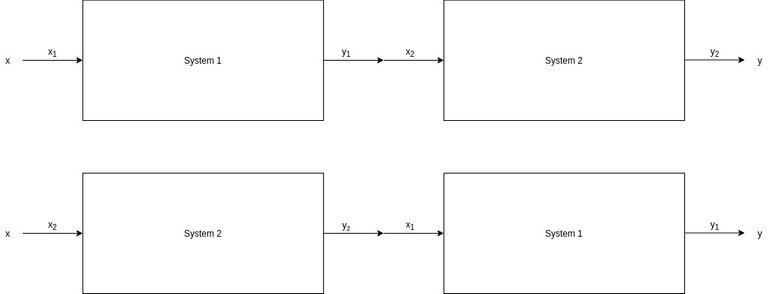[Custom Figure using draw.io]

First of all, let's put two LTI systems in series (also called cascade). Passing the input x through system 1 first and then system 2, or vise versa, results in the same response y. So, when series/cascade interconnection is needed, then the order at which the the individual systems operate on the individual inputs doesn't matter.

Note: Of course x2 = y1 in the top and x1 = y2 in the bottom diagram.

### Parallel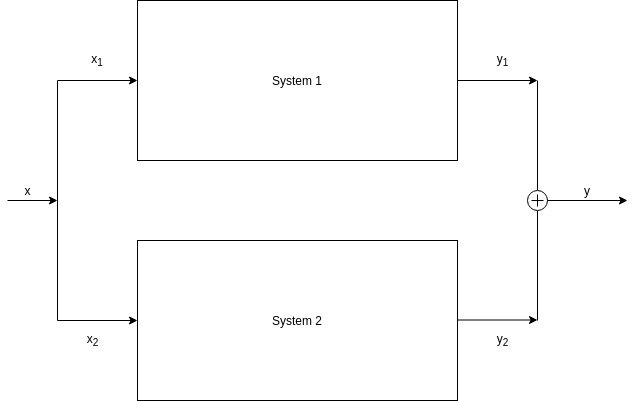[Custom Figure using draw.io]

Next up is parallel interconnection. When the responses of individual LTI systems are added together, in any order, then the total system response will always be the same.

### Feedback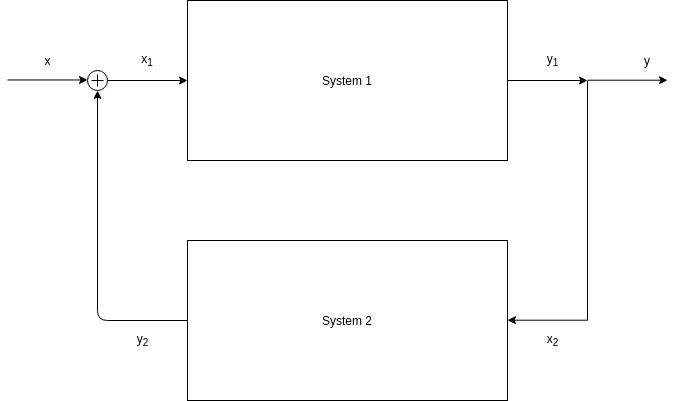[Custom Figure using draw.io]

Another useful interconnection is feedback. Similar to cascading, system 2 gets its input from the output of system 1, and so x2 = y1. But, the input to system 1 is equal to some combination of the system input x and the output y2 of system 2 (x1 = x + y2 in this case). So, the response of system 1 on the input x, is send to another system (the output is of course also the total system response), which in turn gives a feedback response to system 1, changing the input x1 given to system 1, respectively.

This is very useful for implementing any kind of system that involves differential equations, difference equation, integrals. Basically all Physics systems have to be implemented using such LTI Systems with feedback.

## Convolution

Now that interconnection of LTI systems is out of the way, we can head into convolution. So, what exactly is convolution? Well, any system that satisfies both linearity and time-invariance, can be represented in continuous- and discrete-time using combination of two basic signals:

• delayed impulses
• complex exponentials
The resulting representation is called a system convolution.

Later in this series of articles, we will cover in detail how any LTI system can be represented as a linear combination of complex exponentials, through Fourier Analysis.

### Delayed Impulses

Since LTI systems are time-invariant systems, when the response to one impulse at any time is known, then the impulse to any arbitrary time is also known. So, arbitrary sequences of data, can be easily expressed through linear combinations of delayed impulses.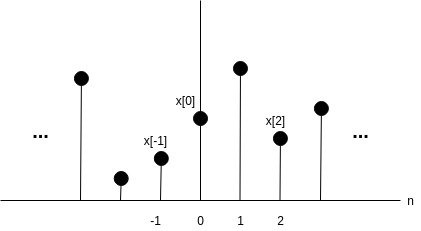[Custom Figure using draw.io]

In discrete-time, the representation of signals is done through the sum of weighted, delayed unit impulses. A unit impulse is the signal δ[n], which is having the value 1 only for one sample, while it remains 0 at any other value. Any delay by k, results in the time-shifted, and so delayed, unit impulse signal δ[n-k]. Multiplying each of the δ[n-k] by some factor (weight) x[k] results in the final weighted, delayed unit impulse x[k]δ[n-k].

So, any discrete-time signal can be represented by the following sum: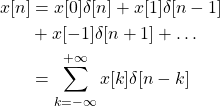### Convolution Sum

Systems can be represented by a weighted sum of delayed unit impulse responses. The unit impulse response is denoted by h[n]. Delaying h[n] by k results in the delayed response h[n-k].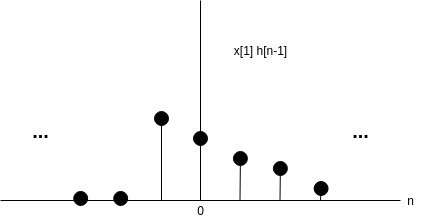[Custom Figure using draw.io]

When a system is linear then any delayed unit impulse δ[n-k] results in the same unit impulse response hk[n], which results in the following sum: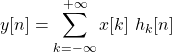For LTI Systems, that also satisfy time-invariance, hk[n] = h[n-k], which gives the following final convolution sum: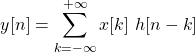In other words, when the system is also time-invariant, then the unit impulse response is no longer sensitive to time.

### Convolution Integral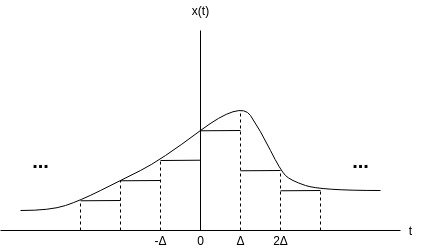[Custom Figure using draw.io]

A continuous-time signal can be approximated by a combination of weighted, delayed rectangular pulses. As the rectangular pulses become increasingly narrow, the representation approaches an integral. Therefore, any general signal can be represented by the following integral: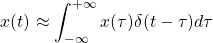For linear systems, δ(t-τ) = hτ(t), and so: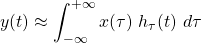For LTI systems, which satisfy time-invariance, hτ(t) = h(t - τ), resulting in the following final convolution integral: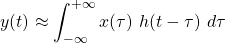### Shortened Representation

In order to skip this quite complex representation, the convolution sum and integral are commonly shortened to: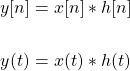## Final words | Next up

And this is actually it for today's post! Till next time!

See Ya!Keep on drifting!

Sort:

Congratulations @drifter1! You have completed the following achievement on the Hive blockchain and have been rewarded with new badge(s) :You distributed more than 88000 upvotes.Your next target is to reach 89000 upvotes.

If you no longer want to receive notifications, reply to this comment with the word `STOP`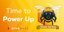Hive Power Up Day - March 1st 2021 - Hive Power DelegationHive Tour Update - Communities